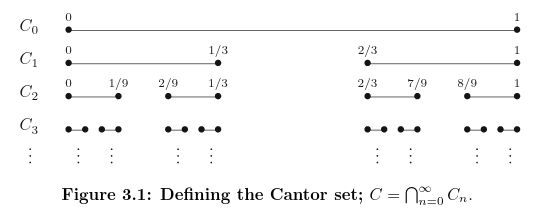Math and science::Analysis

# The Cantor Set

The Cantor set is important for understanding Topology (open and closed sets) and measure.

### Cantor set

Let $$C_0$$ be the closed interval $$[0, 1]$$ and define $$C_1$$ to be the set that results when the open middle third is removed:

[$C_1 = \; C_0 \setminus \; ? = \;\; ?\; \cup \; ?$]

This process continued gives us the Cantor set:

$C = \bigcap_{n=0}^{\infty} C_n$
Or alternatively:
[$C = [0,1] \setminus \big( (\frac{1}{3},\frac{2}{3}) \; \cup \; (?,\,?) \; \cup \; (?,\,?) \; \cup \; ...\big)$ ]### Properties of the Cantor set

Below are some properties of the Cantor set, with explanations on the reverse side.

The cantor set...

• has [zero/finite/infinite] measure
• is [countably/uncountably] infinite
• has dimension of [...]
• is [open/closed/neither/both]
• is [compact/not compact]
• is [perfect/not perfect]
• is [...] dense